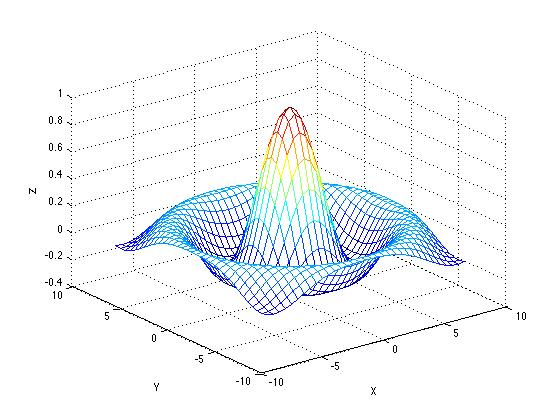# Exercise 26

## Surface plot

Define x and y each over a grid [-8,8] in steps of 0.5.

Define r = $$\sqrt{x^{2} + y^{2}} + 0.00001$$.

Define z = sin(r)/r

Generate the following surface plot:Paul Gribble | fall 2014